Next: Pairing correlations and divergence Up: The Skyrme Hartree-Fock-Bogolyubov equations Previous: The Hartree-Fock-Bogolyubov equations

## Asymptotic properties of the HFB wave functions

The asymptotic properties of the two components of the HFB quasiparticle wave functions, and their dependence on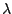and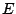, were discussed in Refs. [17,5,18]. Here we complement this discussion by further elements, which pertain mainly to weakly bound systems where the Fermi energyis very small.

Assuming that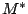=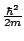, which anyhow is always fulfilled in the asymptotic region, and neglecting for simplicity this trivial mass factor altogether, Eqs. (34) have for large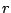the following form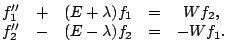(34)

Here we have also neglected all mean-field potentials, assuming that the distance is large enough that they are much smaller than the two characteristic constants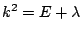and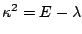. Moreover, we consider the case of small Fermi energy such that there are no discrete HFB states, i.e.,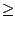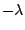. Within such conditions, the only remaining question is whether in the asymptotic region we can neglect the coupling potential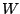.

In order to discuss this question, we note that the coupling terms can be considered as inhomogeneities of the linear equations (35), and therefore, asymptotic solutions have the form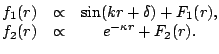(35)

We can now iterate these solutions starting with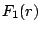=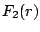=0, which corresponds to neglecting the coupling terms in the zero order. Then, in the first order we see that in the equation for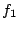the coupling term can still be neglected as compared to the large term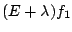. Therefore, to all orders we have=0, and the asymptotic solution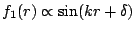defines the inhomogeneity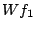of the equation for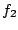.

We arrive here at the conclusion that the asymptotic form ofmay involve two terms and a more detailed analysis is needed before concluding which one dominates. To this end, we note that the coupling potentialdepends on the sum of products of lower and upper components, and therefore has a general form of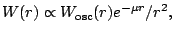(36)

where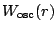is an oscillating function of order 1,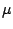is the decay constant, which may characterize another quasiparticle state than the one with energy, and the factor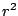comes from the volume element, cf. Eq. (10). We see here that the termin the asymptotic form of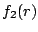vanishes as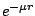, but it is difficult to say which one of the two terms dominates asymptotically. We only note that for small quasiparticle energies the decay constant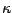is also small and hence the first term is then more likely to dominate.

In order to illustrate the above discussion, we have performed the HFB calculations in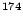Sn, where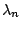=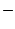0.345MeV and the lowest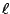=0 quasiparticle state of=0.429MeV (for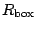=30fm) leads to a very diffused coupling potential with small decay constant. Quasiparticle wave functions corresponding to the four lowest=0 quasiparticle states are shown in Fig. 1. One can see that the asymptotic forms of the second components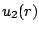of the two lowest quasiparticle states are not affected by the second term, at least up to 30fm. Only the third and fourth states switch at large distances to the oscillating asymptotic forms with a smaller decay constant. This happens at rather large distances where the densities are anyhow very small. Hence the change in the asymptotic properties does not affect any important nuclear observables.

In all cases that we have studied, the practical importance of the second termis negligible. However, its presence precludes simple analytic continuation of the wave functions in the asymptotic region. We would like to stress that the asymptotic forms discussed in this section are numerically stable, and that they are unrelated to the numerical instabilities discussed in Sec. 5.3 below.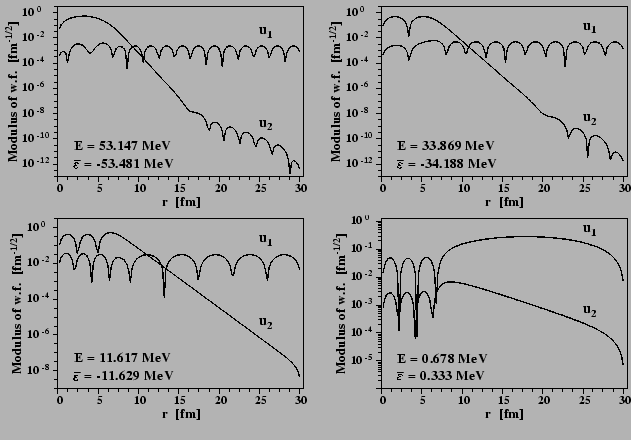Next: Pairing correlations and divergence Up: The Skyrme Hartree-Fock-Bogolyubov equations Previous: The Hartree-Fock-Bogolyubov equations
Jacek Dobaczewski 2005-01-23